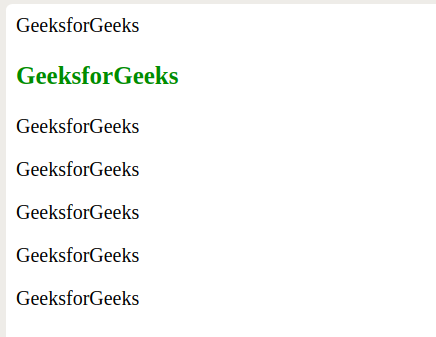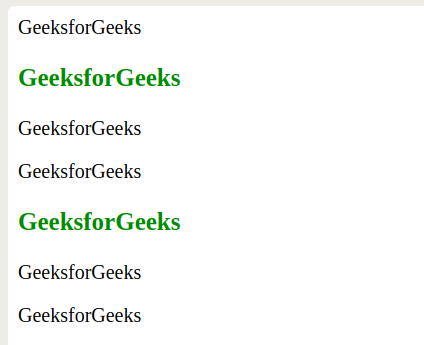# How to get specific number of child elements using CSS?

The :nth-child() in CSS Selector is used to select only those elements that are the nth child, regardless of type, of its parent.

Syntax:

```: nth-child(arg) {
// CSS Property;
}
```

where arg is an argument that represents the pattern for matching elements. It can be number, odd, even or linear equation.

• number: It represents the elements whose position is specified by the argument.
• odd: It represents the elements whose position is odd i.e., 1, 3, 5, etc.
• even: It represents the elements whose position is even i.e, 2, 4, 6, etc.
• linear equation: It represents the elements whose position matches the pattern A*n + B, for every positive integer n. Value of n starts with zero.

Example 1: This example selects the element which is passed as the argument.

 ` ` `<``html``> ` ` `  `<``head``> ` `    ``<``style``> ` `        ``p:nth-child(2) { ` `            ``color: green; ` `            ``font-weight: bold; ` `            ``font-size: 20px; ` `        ``} ` `    `` ` ` ` ` `  `<``body``> ` `    ``<``p``>GeeksforGeeks ` `    ``<``p``>GeeksforGeeks ` `    ``<``p``>GeeksforGeeks ` `    ``<``p``>GeeksforGeeks ` `    ``<``p``>GeeksforGeeks ` `    ``<``p``>GeeksforGeeks ` `    ``<``p``>GeeksforGeeks ` ` ` ` `  `                     `

Output:Example 2: This example selects the even child elements.

 ` ` `<``html``> ` ` `  `<``head``> ` `    ``<``style``> ` `        ``p:nth-child(even) { ` `            ``color: green; ` `            ``font-weight: bold; ` `            ``font-size: 20px; ` `        ``} ` `    `` ` ` ` ` `  `<``body``> ` `    ``<``p``>GeeksforGeeks ` `    ``<``p``>GeeksforGeeks ` `    ``<``p``>GeeksforGeeks ` `    ``<``p``>GeeksforGeeks ` `    ``<``p``>GeeksforGeeks ` `    ``<``p``>GeeksforGeeks ` `    ``<``p``>GeeksforGeeks ` ` ` ` `  `                     `

Output :Example 3: This example selects the odd child elements.

 ` ` `<``html``> ` ` `  `<``head``> ` `    ``<``style``> ` `        ``p:nth-child(odd) { ` `            ``color: green; ` `            ``font-weight: bold; ` `            ``font-size: 20px; ` `        ``} ` `    `` ` ` ` ` `  `<``body``> ` `    ``<``p``>GeeksforGeeks ` `    ``<``p``>GeeksforGeeks ` `    ``<``p``>GeeksforGeeks ` `    ``<``p``>GeeksforGeeks ` `    ``<``p``>GeeksforGeeks ` `    ``<``p``>GeeksforGeeks ` `    ``<``p``>GeeksforGeeks ` ` ` ` `  `                     `

Output :Example 4: This example takes the linear equation as an argument.

 ` ` `<``html``> ` ` `  `<``head``> ` `    ``<``style``> ` `        ``p:nth-child(3n + 2) { ` `            ``color: green; ` `            ``font-weight: bold; ` `            ``font-size: 20px; ` `        ``} ` `    `` ` ` ` ` `  `<``body``> ` `    ``<``p``>GeeksforGeeks ` `    ``<``p``>GeeksforGeeks ` `    ``<``p``>GeeksforGeeks ` `    ``<``p``>GeeksforGeeks ` `    ``<``p``>GeeksforGeeks ` `    ``<``p``>GeeksforGeeks ` `    ``<``p``>GeeksforGeeks ` ` ` ` `  `                     `

Output:My Personal Notes arrow_drop_upI am a quick learner and eager to learn new technologies

If you like GeeksforGeeks and would like to contribute, you can also write an article using contribute.geeksforgeeks.org or mail your article to contribute@geeksforgeeks.org. See your article appearing on the GeeksforGeeks main page and help other Geeks.

Please Improve this article if you find anything incorrect by clicking on the "Improve Article" button below.